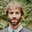# Heating demand calculation with HVAC SystemsDear Forum members,

I am simulating a residential building with Energy Plus and ARCHSIM, and I am completely confused about how to calculate the heat demand.

First I ran a simulation with heating setpoint at 21°C , Infiltration rate 0.15 and natural ventilation with 0.25 ach per hour. The result is quite easy to understand since the "Ideal loads zone total heating energy" matches the "Zone ideal loads air supply total heating energy"

"Zone ideal loads supply air total heating energy" approx =0Wh/m²
"Zone ideal loads supply air total cooling energy"   =0
"Zone ideal loads zone total heating energy"  approx 20kWh/m²
"Zone ideal loads zone total cooling energy"  approx  =0 kWh/m²
"Zone ideal loads outdoor air total heating energy"  approx 20kWh/m²
"Zone ideal loads outdoor air total cooling energy"   =0
"Zone ideal loads heat recovery total heating energy"  =0
"Zone ideal loads heat recovery total heating energy"  =0


So in total 20kWh => outside air heating match the total heating energy

When I involve mechanical ventilation instead of natural to provide me with fresh air (same Infiltration, same heating Point) I get results in 5 out of 8 energy output categories:

"Zone ideal loads supply air total heating energy" approx 15k.24Wh/m²
"Zone ideal loads supply air total cooling energy"   =0
"Zone ideal loads zone total heating energy"  approx 14.79kWh/m²
"Zone ideal loads zone total cooling energy"  approx  8.64kWh/m²
"Zone ideal loads outdoor air total heating energy"  approx 1.35kWh/m²
"Zone ideal loads outdoor air total cooling energy"   =0
"Zone ideal loads heat recovery total heating energy"  approx 1.69kWh/m²
"Zone ideal loads heat recovery total heating energy"  =0


Here If I would add up all categories together it seems like it is too high. I am not sure which of the categories should be taken into the calculation. Anyone can please help on this?